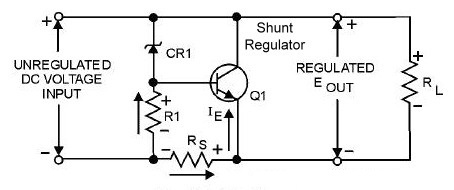## Voltage Regulators

Voltage Regulators:
The circuit which reduces the variations in output DC voltage of an unregulated DC power supply is called as “voltage regulator”.
V.R. = VNL – VFL / VNL
% regulation = V.R. *100 %

Voltage Regulation factors:
Variations in output voltage due to IL :
The output voltage of an unregulated power supply reduces with increase in load current IL, due to the voltage drop across secondary resistance. When the voltage regulator is introduced, the output voltage is made to remain constant in spite of change in IL
Input voltage Vin:
The output voltage of an unregulated power supply increases with increases in input voltage applied to the rectifier. The input voltage the regulator comes from unregulated power supply.
Temperature T:
The output voltage of an unregulated power supply changes with change in temperature because of characteristics of electronic devices used in rectifiers, filters & regulators are temperature dependent.

#### Zener Diode as a Voltage Regulator or (shunt voltage regulator):

Construction Of Shunt Voltage Regulator
• The zener diode connected parallel with the load therefore it also known as shunt voltage regulator.
• The series resistance R is connected in series with the zener diode to limit the zener current.
• The Vo is taken across RL & zener diode of zener voltage Vz is reversely connected by using unregulated DC voltage.
• As we know the reverse bias characteristics of zener diode at breakdown voltage zener current increases rapidly & it produce constant voltage across diode.
• By clearly observe that the zener diode is used as voltage regulator in reverse bias condition.

Operation:
By connecting variable DC voltage to circuit, zener current will flows to the circuit through R resistor.
For proper operation Vin should be greater than breakdown voltage Vz i.e.Vz=Vo.
The input current iR is given by,

IR= (Vin - Vz) / R   -------------------i
But IR = Iz + Io & Iz = IR – Io                 ----------------------ii
From eq. ii as load current increases the load current decreases so, the input current remains constant.
By using KVL,
Vo = Vin – IR . R
Thus the zener diode acts as a voltage regulator & fixed voltage maintained across RL.

• Very simple circuit.
• Only 2 or 3 components are required.
• It has low cost.

• Poor voltage regulation.
• Poor efficiency
• Not suitable for large currents.
• Output resistance is high.
• Output voltage is not adjustable

.Application:
• In emitter follower regulator.
• Used with small load range.

#### Transistorized Series Voltage Regulator:Transistorized Series Voltage Regulator

#### Operating Principle

• The Q1 transistor acts as a control element because it connected in series with the load, hence it is called as a series regulator. It also called as “Emitter Follower Regulator”
• Since Q1 is an npn transistor, Vo is found as:

• The response of the pass-transistor to a change in load resistance as follows
• Since the Zener voltage is constant, the increase in Vo causes VBE to decrease.
• The decrease in VBE reduces conduction through the pass- transistor, so load current decreases.
• This offsets the increase in load resistance, and a relatively constant load voltage is maintained.

• Very low output resistance.
• Less ripple at output terminal.
• Voltage regulation is better than shunt.
• Good efficiency.
• Output voltage is constant & it is independent of temperature.

• Due to excessive power dissipation transistor may damage.
• Output voltage is not adjustable.
• For large currents voltage regulation is not so good.

Applications:
• Used as fixed voltage regulators.
• Used as voltage source with low output current sourcing capacity.
• Used in laboratories.

Transistorized Shunt Voltage Regulator:Transistorized Shunt Voltage Regulator

• The control element is a transistor, in parallel with the load. While, the resistor, RS, is in series with the load.
• The operation of the transistor shunt regulator is similar to that of the transistor series regulator, except that regulation is achieved by controlling the current through the parallel transistor.
•  Resistor RS drops the unregulated voltage depends on current supplied to load RL.
• Voltage across the load is set by zener diode and transistor base-emitter voltage.
• If RL decrease, a reduced drive current to base of Q1  shunting less collector current.
•  Load current, IL is larger, maintaining the regulated voltage across load.
• The output voltage to the load is: Voltage across the load is set by the Zener diode voltage and the transistor base-emitter voltage.

• Good voltage regulation.
• Good efficiency for small load currents.
• Output voltage is constant.
• Output resistance Ro is of low value.

• Power dissipation is taking place in series resistor R.
•  High output resistance.
• Poor efficiency for large loads.

Applications:
• Used for low power application.
• Used in less expensive applications.